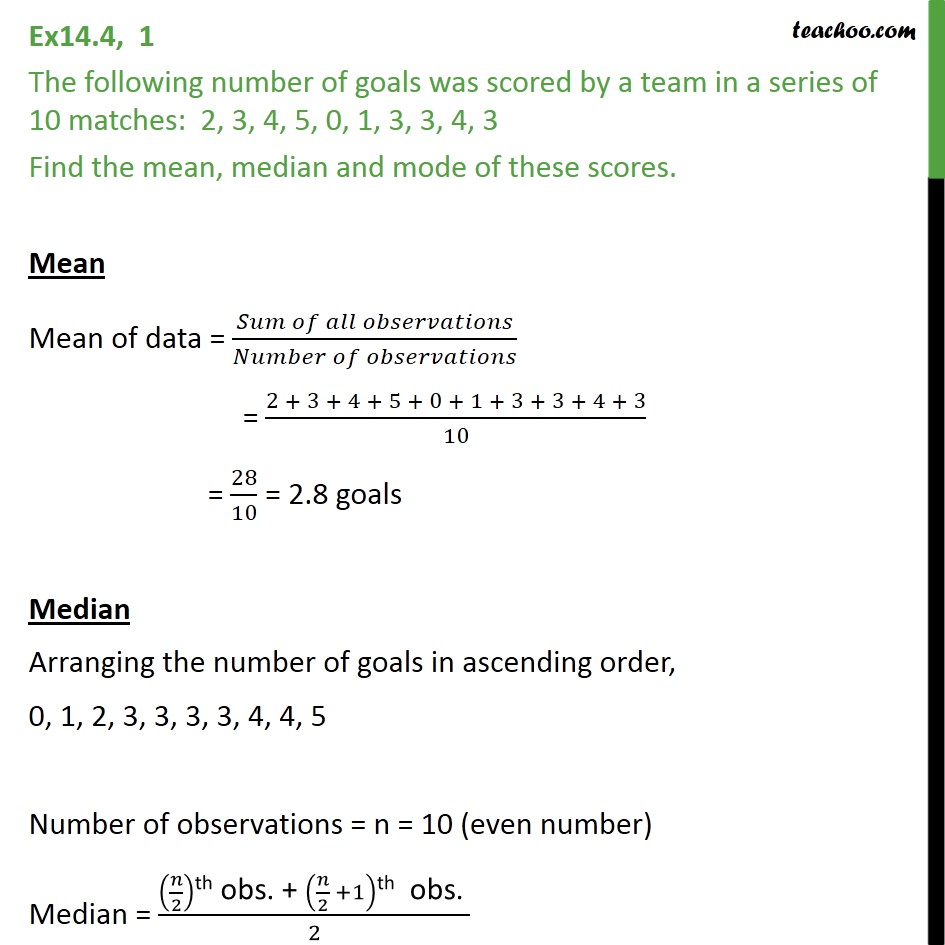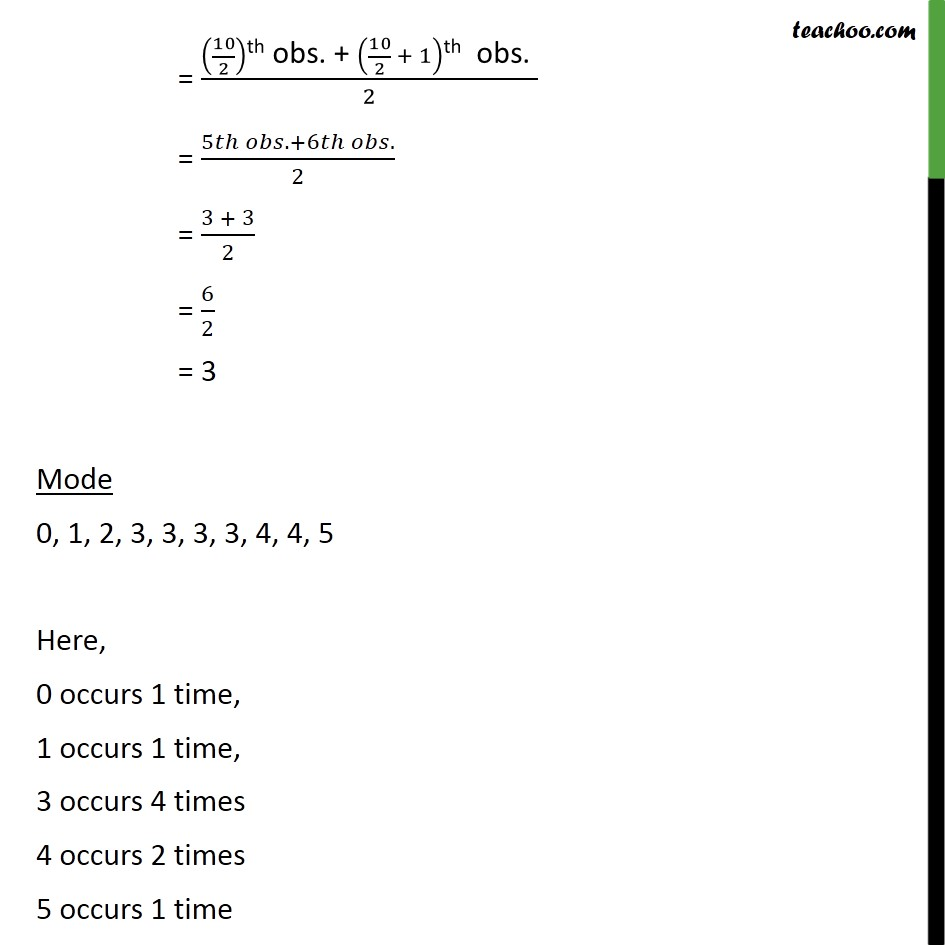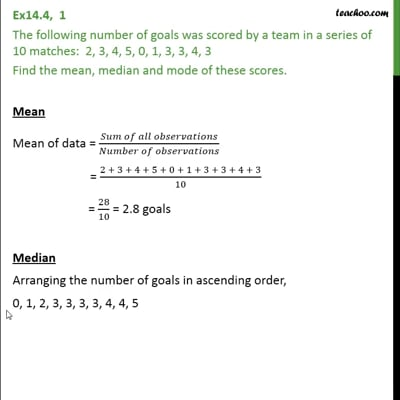Chapter 14 Class 9 Statistics

Class 9
Important Questions for Exam - Class 9This video is only available for Teachoo black users

Introducing your new favourite teacher - Teachoo Black, at only ₹83 per month

### Transcript

Ex14.4, 1 The following number of goals was scored by a team in a series of 10 matches: 2, 3, 4, 5, 0, 1, 3, 3, 4, 3 Find the mean, median and mode of these scores. Mean Mean of data = (𝑆𝑢𝑚 𝑜𝑓 𝑎𝑙𝑙 𝑜𝑏𝑠𝑒𝑟𝑣𝑎𝑡𝑖𝑜𝑛𝑠)/(𝑁𝑢𝑚𝑏𝑒𝑟 𝑜𝑓 𝑜𝑏𝑠𝑒𝑟𝑣𝑎𝑡𝑖𝑜𝑛𝑠) = (2 + 3 + 4 + 5 + 0 + 1 + 3 + 3 + 4 + 3)/10 = 28/10 = 2.8 goals Median Arranging the number of goals in ascending order, 0, 1, 2, 3, 3, 3, 3, 4, 4, 5 Number of observations = n = 10 (even number) Median = (𝑛/2)"th obs. + " (𝑛/2 +1)"th obs. " /2 = (10/2)"th obs. + " (10/2 + 1)"th obs. " /2 = (5𝑡ℎ 𝑜𝑏𝑠.+6𝑡ℎ 𝑜𝑏𝑠.)/2 = (3 + 3)/2 = 6/2 = 3 Mode 0, 1, 2, 3, 3, 3, 3, 4, 4, 5 Here, 0 occurs 1 time, 1 occurs 1 time, 3 occurs 4 times 4 occurs 2 times 5 occurs 1 time Since 3 occurs maximum number of times Mode = 3